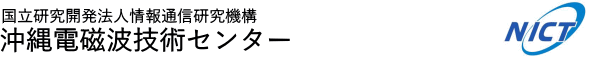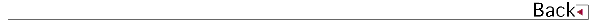# 2008\$BG/EY(B (2008.04\$B!A(B2009.03)

## \$B8&5fO@J8(B

• W. L. Oliver, \$B@nB<(B \$B@?<#(B, \$B?;0lO:(B, The causes of mid-latitude F layer behavior, JOURNAL OF GEOPHYSICAL RESEARCH, Vol.113, doi:10.1029/2007JA012590, 2008.
• \$BF#Ln(B \$B5AG7(B, \$B:4F#(B \$B@5pJsEAAw\$N\$?\$a\$N%X%j%3%W%?!<1R@1DL?.5;=Q\$N3+H/(B, \$BEE;R>pJsDL?.3X2q(B \$BO@J8;o(BB, Vol.J91-B, No.12, P1611-1619, 2008.

## \$B<}O?O@J8(B

• \$BFg0f(B \$B>O;L(B, \$BG_86(B \$B=SI'(B, \$B>>2,(B \$B7z;V(B, \$B>.NS(B \$BC#<#(B, \$B:4C](B \$B@?(B, \$B1:DM(B \$B@6Jv(B, \$B9R6u5!Ek:\Fs<~GH(BSAR\$B\$K\$h\$k3\$LL;6MpCGLL@Q\$NIw8~0MB8@-2r@O(B, Dependency of radar cross section of ocean surface on wind direction analyzed by using an airborne SAR, \$BEE;R>pJsDL?.3X2q(B \$B1'Ch!&9R9T%(%l%/%H%m%K%/%98&5f2q(B, SANE2008, P19-23, 2008.
• \$B:4C](B \$B@?(B, \$B=P@\$(B \$B\$f\$+\$j(B, \$BCf@n(B \$B>!9-(B, \$B;yEg(B \$B@50lO:(B, \$B:4F#(B \$B?82p(B, Ocean Surface Observation by C-band Polarimetric Weather Radar in Okinawa Island, IEEE Geoscience and Remote Sensing Symposium 2008 (IGARSS 2008), 2008IEEE, No.I, P402-404, 2008.
• \$BJ!CO!!0l(B, \$BKc@8M:0lO:(B, \$BC]Be>O?M(B, \$B>.>>M4Je(B, \$B:4C](B \$B@?(B, Extraction of area averaged urban parameters from POLSAR measurement, IEEE Geoscience and Remote Sensing Symposium 2008 (IGARSS 2008), 2008IEEE, No.IV, P1241-1244, 2008.
• \$B4X_7(B \$B?.Li(B, \$B@nB<(B \$B@?<#(B, \$B1J0f(B \$B@6Fs(B, \$B:4C](B \$B@?(B, \$BCf@n(B \$B>!9-(B, 400MHz\$BBS%&%#%s%I%W%m%U%!%\$%i\$rMQ\$\$\$?1+E)N37BJ,I[\$N?dDj(B, Estimation of raindrop size distribution by using 400MHz-band wind profiler radar, \$BF|K\N.BNNO3X2q!!G/2q(B2008, No.15041, P1-4, 2008.

## \$B8&5f;qNA(B

• \$B:4C](B \$B@?(B, "JAPAN NATIONAL COMMITTEE FOR RADIO SCIENCE REPORT (Nov. 2004 - Oct. 2007)(Section\$B!K(BF2 Remote Sensing", Vol.RPT08, No.F08, P14-20, 2008.

• \$B

## \$B0lHL8}F,H/I=(B

• \$Bo4QB,(B, \$BF|K\5\$>]3X2q(B2008\$BG/EY=U4|Bg2q(B, P240, 2008.
• \$B4X_7(B \$B?.Li(B, \$B@nB<(B \$B@?<#(B, \$B1J0f(B \$B@6Fs(B, \$B:4C](B \$B@?(B, \$BCf@n(B \$B>!9-(B, \$B1+E)N37BJ,I[4QB,\$N\$?\$a\$N%&%#%s%I%W%m%U%!%\$%i9;@5K!(B, \$BF|K\5\$>]3X2q(B \$B=U5(Bg2q(B, No.242, P404, 2008.
• \$B:4C](B \$B@?(B, \$B=P@\$\$f\$+\$j(B, \$BCf@n(B \$B>!9-(B, \$B;yEg(B \$B@50lO:(B, \$B:4F#(B \$B?82p(B, C-band\$B2-FlJPGH9_1+%l!<%@\$K\$h\$k3\$LL4QB,(B(2)\$B!'JPGH4QB,\$H%I%C%W%i4QB,7k2L(B, Ocean surface observation by C-band polarimetric weather radar in Okinawa (2): evaluation of cross-polarization signals and Doppler signals, \$BF|K\%j%b!<%H%;%s%7%s%03X2q!!Bh(B44\$B2s3X=Q9V1i2q(B, No.B15, P65-66, 2008.
• \$B:4F#(B \$BM[2p(B, \$BCfB<(B \$BBn;J(B, \$BDEED(B \$BIRN4(B, \$B:4F#(B \$B?82p(B, \$B:4C](B \$B@?(B, \$BB<;3(B \$BBY7<(B, \$B>.7?%i%^%s%i%\$%@!<\$K\$h\$k2-Fl0!G.BS0h2x5\$J,I[\$N4QB,(B, Observation of water vapor distributionin the lower troposphere over Okinawa with a small Raman lidar, \$BF|K\CO5eOG@12J3XO"9g(B2008\$BG/Bg2q(B, E115-003, 2008.
• \$BB<;3(B \$BBY7<(B, \$B4d0f9(FA(B, \$B@nB<(B \$B@?<#(B, \$B4X_7(B \$B?.Li(B, \$B@P0f(B \$B>;7{(B, \$B>o>>(B \$BE8=<(B, Gernot Hassenpflug, \$B?eC+(B \$B9LJ?(B, NICT \$B6-3&AX4QB,%W%m%8%'%/%H\$N8=>u(B, \$BCO5eEE<'5\$!&CO5eOG@17w3X2q(B \$BAm2q5Z\$S9V1i2q(B (SGEPSS), 2008.
• \$B:4C](B \$B@?(B, NICT\$B2-Fl0!G.BS7WB,5;=Q%;%s%?!<\$N>R2p(B, \$B%\$%s%?!<%M%C%H%3%s%U%!%l%s%9(B2008\$B!JFCJL9V1i!K(B, P1130, 2008.
• \$B:4F#(B \$B?82p(B, \$B8EK\(B \$B=_0l(B, \$Be(B \$B:L(B, \$BDEED(B \$BIRN4(B, \$B1J0f(B \$B@6Fs(B, \$BB<;3(B \$BBY7<(B, 400MHz\$BBS(BWPR/RASS\$B\$G4QB,\$5\$l\$?BPN.6-3&AX\$NH/C#(B, \$BF|K\5\$>]3X2q(B2008\$BG/EY=)4|Bg2q(B, C101, 2008.
• \$B:4C](B \$B@?(B, \$B=P@\$\$f\$+\$j(B, \$BCf@n>!9-(B, \$B0f8}=SIW(B, \$B2-FlJPGH9_1+%l!<%@(B(COBRA)\$B\$K\$h\$k3\$LL;6Mp\$NB,Dj(B, URSI-F\$B8&5f2q(B, No.530-3, 2008.
• \$B:4F#(B \$B?82p(B, \$B8EK\(B \$B=_0l(B, \$Be(B \$B:L(B, \$BDEED(B \$BIRN4(B, \$B1J0f(B \$B@6Fs(B, \$BB<;3(B \$BBY7<(B, 400MHz\$BBS%&%#%s%I%W%m%U%!%\$%i(B/RASS\$B\$G4QB,\$5\$l\$?BPN.6-3&AX\$NH/C#(B,\$B!V2-Fl\$N5\$>]!&5\$8u!&3\$>]\$N4QB,E*8&5f!W\$K4X\$9\$k6&F18&5f=82q!J(BNICT-HyARC \$BJ?@.(B20\$BG/EY6&F18&5f=82q!K(B, 2009.
• \$B@nH*(B \$BBsLp(B, \$B;0>e(B \$B:L(B, \$B8EK\(B \$B=_0l(B, \$B:4F#(B \$B?82p(B, \$B1J0f(B \$B@6Fs(B, \$BBg59L#4QB,=j\$K\$*\$1\$k(BRASS\$B4QB,%G!<%?F12=]!&5\$8u!&3\$>]\$N4QB,E*8&5f!W\$K4X\$9\$k6&F18&5f=82q!J(BNICT-HyARC \$BJ?@.(B20\$BG/EY6&F18&5f=82q!K(B, 2009.
• \$BCfB<(B \$B7r<#(B, \$B?9K\(B \$B>Bg(BHyARC\$B\$H\$N6&F18&5f(B, NICT\$B2-Fl\$HL>Bg(BHyARC\$B\$H\$N6&F18&5f(B, \$B!V2-Fl\$N5\$>]!&5\$8u!&3\$>]\$N4QB,E*8&5f!W\$K4X\$9\$k6&F18&5f=82q!J(BNICT-HyARC \$BJ?@.(B20\$BG/EY6&F18&5f=82q!K(B, 2009.
• \$B1J0f(B \$B@6Fs(B, \$B:4F#(B \$B?82p(B, \$B8EK\(B \$B=_0l(B, \$B4X_7(B \$B?.Li(B, \$B:4C](B \$B@?(B, \$BBg59L##4#0#0(BMH\$B#zBS#W#P#R!?#R#A#S#S\$N1?MQ>u67\$K\$D\$\$\$F(B, , HyARC-NICT\$B6&F18&5f=82q(B, No.5, P15-17, 2009.
• \$B:4F#(B \$B?82p(B, \$B8EK\(B \$B=_0l(B, \$BDEED(B \$BIRN4(B, \$B1J0f(B \$B@6Fs(B, \$BB<;3(B \$BBY7<(B, 400MHz\$BBS(BWPR/RASS\$B\$rMQ\$\$\$?Bg5\$6-3&AX\$N4QB,(B, \$BBh#12s(BRASS\$B8&5f2q(B, 2009.
• \$B@nB<(B \$B@?<#(B, \$B4X_7(B \$B?.Li(B, \$B1J0f(B \$B@6Fs(B, \$B:4C](B \$B@?(B, \$BCf@n(B \$B>!9-(B, 400MHz\$BBS%&%#%s%I%W%m%U%!%\$%i\$K\$h\$k1+E)N37BJ,I[?dDj\$N<+F02=(B, , \$BF|K\5\$>]3X2q(B \$B=U5(Bg2q(B, 2008.
• \$B@nB<(B \$B@?<#(B, MF\$B%l!<%@!<4QB,\$N>R2p(B, \$BCf4V7w!&G.7w!&EEN%7w8&5f2q(B, 2008.
• \$B:4C](B \$B@?(B, \$B=P@\$\$f\$+\$j(B, \$BCf@n(B \$B>!9-(B, \$B@nB<(B \$B@?<#(B, \$B0f8}(B \$B=SIW(B, COBRA\$B\$K\$h\$k3\$LL\$N;6MpFC@-7WB,(B(II), \$B!V2-Fl\$N5\$>]!&5\$8u!&3\$>]\$N4QB,E*8&5f!W\$K4X\$9\$k6&F18&5f=82q(B, 2009.
• \$B@nB<(B \$B@?<#(B, \$B:4C](B \$B@?(B, \$BCf@n(B \$B>!9-(B, \$B2VEZ(B \$B90(B, \$B9b66(B \$BD*9((B, \$B:4F#(B \$B?82p(B, \$B0f8}(B \$B=SIW(B, COBRA\$B%*!<%P!<%[!<%k\$K\$D\$\$\$F(B, HyARC-NICT\$B8&5f=82q(B, 2009.
• \$B@nB<(B \$B@?<#(B, \$B:4C](B \$B@?(B, \$B1J0f(B \$B@6Fs(B, \$B2VEZ(B \$B90(B, \$B:4F#(B \$B?82p(B, \$BL1ED(B \$B@2Li(B, \$B8E_7(B \$BJ89>(B, \$BCfB<(B \$B7r<#(B, JGN2plus\$B2s@~\$rMQ\$\$\$?BgMFNL%G!<%?\$N9-0hJ,;62r@O(B, HyARC-NICT\$B8&5f=82q(B, 2009.

## 1990\$B!A(B1991\$B!d(B1992\$B!d(B1993\$B!d(B1994\$B!d(B1995\$B!d(B1996\$B!d(B1997\$B!d(B1998\$B!d(B 1999\$B!d(B2000\$B!d(B2001\$B!d(B2002\$B!d(B2003\$B!d(B2004\$B!d(B2005\$B!d(B2006\$B!d(B2007\$B!d(B 2008\$B!d(B2009\$B!d(B2010\$B!d(B2011\$B!d(B2012\$B!d(B2013\$B!d(B2014\$B!d(B2015\$B!d(B2016\$B!d(B2017